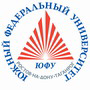Russian©  M. E. Abramyan (Southern Federal University, Shenzhen MSU-BIT University), 1998–2023

 Main Tasks Examples PT for MPI-2

 Overview Input-output operations Task groups Begin Integer Boolean If Case For While Series Proc Func Minmax Array Matrix String File Text Param Recur Dynamic Dynamic (obj) Tree Tree (obj)

# Logical expressions

All tasks in this group require determining the proposition as True or False. All numbers with fixed amount of digits (for example, two-digit number, three-digit number and so on) are assumed to be positive integers.

Boolean1°. Given integer A, verify the following proposition: "The number A is positive".

Boolean2°. Given integer A, verify the following proposition: "The number A is odd".

Boolean3°. Given integer A, verify the following proposition: "The number A is even".

Boolean4°. Given two integers A and B, verify the following proposition: "The inequalities A > 2 and B ≤ 3 both are fulfilled".

Boolean5°. Given two integers A and B, verify the following proposition: "The inequality A ≥ 0 is fulfilled or the inequality B < −2 is fulfilled".

Boolean6°. Given three integers A, BC, verify the following proposition: "The double inequality A < B < C is fulfilled".

Boolean7°. Given three integers A, BC, verify the following proposition: "The number B is between A and C".

Boolean8°. Given two integers A and B, verify the following proposition: "Each of the numbers A and B is odd".

Boolean9°. Given two integers A and B, verify the following proposition: "At least one of the numbers A and B is odd".

Boolean10°. Given two integers A and B, verify the following proposition: "Exactly one of the numbers A and B is odd".

Boolean11°. Given two integers A and B, verify the following proposition: "The numbers A and B have equal parity".

Boolean12°. Given three integers A, BC, verify the following proposition: "Each of the numbers A, BC is positive".

Boolean13°. Given three integers A, BC, verify the following proposition: "At least one of the numbers A, BC is positive".

Boolean14°. Given three integers A, BC, verify the following proposition: "Exactly one of the numbers A, BC is positive".

Boolean15°. Given three integers A, BC, verify the following proposition: "Exactly two of the numbers A, BC are positive".

Boolean16°. Given a positive integer, verify the following proposition: "The integer is a two-digit even number".

Boolean17°. Given a positive integer, verify the following proposition: "The integer is a three-digit odd number".

Boolean18°. Verify the following proposition: "Among three given integers there is at least one pair of equal ones".

Boolean19°. Verify the following proposition: "Among three given integers there is at least one pair of opposite ones".

Boolean20°. Given a three-digit integer, verify the following proposition: "All digits of the number are different".

Boolean21°. Given a three-digit integer, verify the following proposition: "All digits of the number are in ascending order".

Boolean22°. Given a three-digit integer, verify the following proposition: "All digits of the number are in ascending or descending order".

Boolean23°. Given a four-digit integer, verify the following proposition: "The number is read equally both from left to right and from right to left".

Boolean24°. Three real numbers A, BC are given (A is not equal to 0). By means of a discriminant D = B2 − 4·A·C, verify the following proposition: "The quadratic equation A·x2 + B·x + C = 0 has real roots".

Boolean25°. Given two nonzero real numbers x, y, verify the following proposition: "The point with coordinates (xy) is in the second coordinate quarter".

Boolean26°. Given two nonzero real numbers x, y, verify the following proposition: "The point with coordinates (xy) is in the fourth coordinate quarter".

Boolean27°. Given two nonzero real numbers x, y, verify the following proposition: "The point with coordinates (xy) is in the second or third coordinate quarter".

Boolean28°. Given two nonzero real numbers x, y, verify the following proposition: "The point with coordinates (xy) is in the first or third coordinate quarter".

Boolean29°. Given real numbers x, y, x1y1, x2y2, verify the following proposition: "The point (xy) is inside of the rectangle whose left top vertex is (x1y1), right bottom vertex is (x2y2), and sides are parallel to coordinate axes".

Boolean30°. Given three integers a, bc that are the sides of a triangle, verify the following proposition: "The triangle with sides a, bc is equilateral".

Boolean31°. Given three integers a, bc that are the sides of a triangle, verify the following proposition: "The triangle with sides a, bc is isosceles".

Boolean32°. Given three integers a, bc that are the sides of a triangle, verify the following proposition: "The triangle with sides a, bc is a right triangle".

Boolean33°. Given three integers a, bc, verify the following proposition: "A triangle with the sides a, bc exists".

Boolean34°. Given coordinates x, y of a chessboard square (as integers in the range 1 to 8), verify the following proposition: "The chessboard square (xy) is white". Note that the left bottom square (1, 1) is black.

Boolean35°. Given coordinates x1, y1, x2y2 of two chessboard squares (as integers in the range 1 to 8), verify the following proposition: "Both of the given chessboard squares have the same color".

Boolean36°. Given coordinates x1, y1, x2y2 of two chessboard squares (as integers in the range 1 to 8), verify the following proposition: "A rook can move from one square to another during one turn".

Boolean37°. Given coordinates x1, y1, x2y2 of two chessboard squares (as integers in the range 1 to 8), verify the following proposition: "A king can move from one square to another during one turn".

Boolean38°. Given coordinates x1, y1, x2y2 of two chessboard squares (as integers in the range 1 to 8), verify the following proposition: "A bishop can move from one square to another during one turn".

Boolean39°. Given coordinates x1, y1, x2y2 of two chessboard squares (as integers in the range 1 to 8), verify the following proposition: "A queen can move from one square to another during one turn".

Boolean40°. Given coordinates x1, y1, x2y2 of two chessboard squares (as integers in the range 1 to 8), verify the following proposition: "A knight can move from one square to another during one turn".Designed byM. E. Abramyan and V. N. Braguilevsky Last revised:01.01.2023